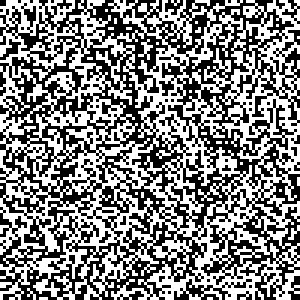# Ising model simulation

The Ising model is a model used to represent magnetic dipole moments in statistical physics. Physical details are on the Wikipedia page, but what is interesting is that it follows a complex probability distribution on a lattice, where each site can take the value +1 or -1.## Mathematical definition

We have a lattice $$\Lambda$$ consisting of sites $$k$$. For each site, there is a moment $$\sigma_k \in \{ -1, +1 \}$$. $$\sigma = (\sigma_k)_{k\in\Lambda}$$ is called the configuration of the lattice.

The total energy of the configuration is given by the Hamiltonian $H(\sigma) = -\sum_{i\sim j} J_{ij}\, \sigma_i\, \sigma_j,$ where $$i\sim j$$ denotes neighbours, and $$J$$ is the interaction matrix.

The configuration probability is given by: $\pi_\beta(\sigma) = \frac{e^{-\beta H(\sigma)}}{Z_\beta}$ where $$\beta = (k_B T)^{-1}$$ is the inverse temperature, and $$Z_\beta$$ the normalisation constant.

For our simulation, we will use a constant interaction term $$J > 0$$. If $$\sigma_i = \sigma_j$$, the probability will be proportional to $$\exp(\beta J)$$, otherwise it would be $$\exp(\beta J)$$. Thus, adjacent spins will try to align themselves.

## Simulation

The Ising model is generally simulated using Markov Chain Monte Carlo (MCMC), with the Metropolis-Hastings algorithm.

The algorithm starts from a random configuration and runs as follows:

1. Select a site $$i$$ at random and reverse its spin: $$\sigma'_i = -\sigma_i$$
2. Compute the variation in energy (hamiltonian) $$\Delta E = H(\sigma') - H(\sigma)$$
3. If the energy is lower, accept the new configuration
4. Otherwise, draw a uniform random number $$u \in ]0,1[$$ and accept the new configuration if $$u < \min(1, e^{-\beta \Delta E})$$.

## Implementation

The simulation is in Clojure, using the Quil library (a Processing library for Clojure) to display the state of the system.

This post is “literate Clojure”, and contains core.clj. The complete project can be found on GitHub.

(ns ising-model.core
(:require [quil.core :as q]
[quil.middleware :as m]))

The application works with Quil’s functional mode, with each function taking a state and returning an updated state at each time step.

The setup function generates the initial state, with random initial spins. It also sets the frame rate. The matrix is a single vector in row-major mode. The state also holds relevant parameters for the simulation: $$\beta$$, $$J$$, and the iteration step.

(defn setup [size]
"Setup the display parameters and the initial state"
(q/frame-rate 300)
(q/color-mode :hsb)
(let [matrix (vec (repeatedly (* size size) #(- (* 2 (rand-int 2)) 1)))]
{:grid-size size
:matrix matrix
:beta 10
:intensity 10
:iteration 0}))

Given a site $$i$$, we reverse its spin to generate a new configuration state.

(defn toggle-state [state i]
"Compute the new state when we toggle a cell's value"
(let [matrix (:matrix state)]
(assoc state :matrix (assoc matrix i (* -1 (matrix i))))))

In order to decide whether to accept this new state, we compute the difference in energy introduced by reversing site $$i$$: $\Delta E = J\sigma_i \sum_{j\sim i} \sigma_j.$

The filter some? is required to eliminate sites outside of the boundaries of the lattice.

(defn get-neighbours [state idx]
"Return the values of a cell's neighbours"
[(get (:matrix state) (- idx (:grid-size state)))
(get (:matrix state) (dec idx))
(get (:matrix state) (inc idx))
(get (:matrix state) (+ (:grid-size state) idx))])

(defn delta-e [state i]
"Compute the energy difference introduced by a particular cell"
(* (:intensity state) ((:matrix state) i)
(reduce + (filter some? (get-neighbours state i)))))

We also add a function to compute directly the hamiltonian for the entire configuration state. We can use it later to log its values across iterations.

(defn hamiltonian [state]
"Compute the Hamiltonian of a configuration state"
(- (reduce + (for [i (range (count (:matrix state)))
j (filter some? (get-neighbours state i))]
(* (:intensity state) ((:matrix state) i) j)))))

Finally, we put everything together in the update-state function, which will decide whether to accept or reject the new configuration.

(defn update-state [state]
"Accept or reject a new state based on energy
difference (Metropolis-Hastings)"
(let [i (rand-int (count (:matrix state)))
new-state (toggle-state state i)
alpha (q/exp (- (* (:beta state) (delta-e state i))))]
;;(println (hamiltonian new-state))
(update (if (< (rand) alpha) new-state state)
:iteration inc)))

The last thing to do is to draw the new configuration:

 (defn draw-state [state]
"Draw a configuration state as a grid"
(q/background 255)
(let [cell-size (quot (q/width) (:grid-size state))]
(doseq [[i v] (map-indexed vector (:matrix state))]
(let [x (* cell-size (rem i (:grid-size state)))
y (* cell-size  (quot i (:grid-size state)))]
(q/no-stroke)
(q/fill
(if (= 1 v) 0 255))
(q/rect x y cell-size cell-size))))
;;(when (zero? (mod (:iteration state) 50)) (q/save-frame "img/ising-######.jpg"))
)

And to reset the simulation when the user clicks anywhere on the screen:

(defn mouse-clicked [state event]
"When the mouse is clicked, reset the configuration to a random one"
(setup 100))
(q/defsketch ising-model
:title "Ising model"
:size [300 300]
:setup #(setup 100)
:update update-state
:draw draw-state
:mouse-clicked mouse-clicked
:features [:keep-on-top :no-bind-output]
:middleware [m/fun-mode])

## Conclusion

The Ising model is a really easy (and common) example use of MCMC and Metropolis-Hastings. It allows to easily and intuitively understand how the algorithm works, and to make nice visualizations!